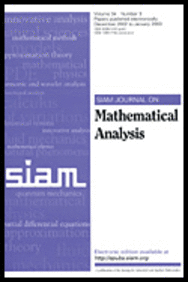Paper of Bevan and Deane on nonlinear elasticity appears in SIAM J Math AnalysisThe paper “A calibration method for estimating critical cavitation loads from below in three-dimensional nonlinear elasticity“, co-authored by Jonathan Bevan and Jonathan Deane, has been published in the SIAM Journal of Mathematical Analysis.  A link to the published electronic version is here. In the paper they give an explicit sufficient condition for the affine lambda-map to be the global energy minimizer of a general class of elastic stored-energy functionals in three space dimensions. The function space setting is such that cavitating (i.e., discontinuous) deformations are admissible. In the language of the calculus of variations, the condition ensures the quasiconvexity of the stored energy functional.  The Bevan-Deane approach relies on arguments involving null Lagrangians (in this case, affine combinations of the minors of 3 by 3 matrices), on previous work [J. Bevan and C. Zeppieri, Calc. Var. Partial Differential Equations, 55 (2015), pp. 1–25], and on a careful numerical treatment to make the calculation of certain constants tractable.